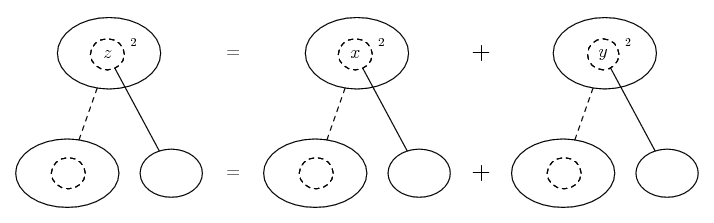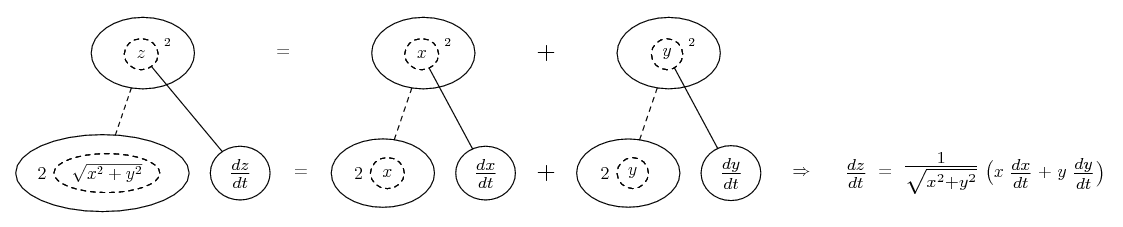# Thread: Related Rates

1. ## Related Rates

An air traffic controller spots two airplanes at the same altitude converging to a point as they fly at right angles to each other. One airplane is 150 miles from the point and has a speed of 450 miles per hour. The other is 200 miles from the point and has a speed of 600 miles per hour.

At what rate is the distance between the planes changing?

- I'm completely lost on this question. I don't know how to proceed.

2. One way is to notice we have all the bits for Pythagoras...So differentiate implicitly, using...... the chain rule, where straight continuous lines differentiate downwards (integrate up) with respect to the main variable (in this case time), and the straight dashed line similarly but with respect to the dashed balloon expression (the inner function of the composite which is subject to the chain rule).

Spoiler:Then sub in the distances and speeds.

__________________________________________

Don't integrate - balloontegrate!

Balloon Calculus: Gallery

Balloon Calculus Drawing with LaTeX and Asymptote!

#### Search Tags

rates, related